# Percentage Problems With Solutions

Percentage Problems With Solutions, deals with various concepts which are as under:-

• Finding a number, whose percentage value is given
• Finding what percentage of a given term is another term
• Net Increase or Decrease Percentage in a Number
• Finding a number when its decreased percentage is given
• Finding a number when its increased percentage is given
• Salary saved

Percentage Problems With Solutions – Finding a number, whose percentage value is given

Question 1:

If 30 % of a number is 60, find the number?

Solution:

We can form a linear equation to express the given statement and then arrive at the number

Let the required number be x

We are given that

30 % of x is 60

or in other words

30/100 × x = 60

On cross multiplication, we get

x = (60 × 100)/30

x = 200

Hence, the number is 200

#### Percentage Problems With Solutions – Finding what percentage of a given term is another term

Question 2:

What percent of 20 litres is 10 litres ?

Solution:

Let a % of 20 litres = 10 litres

Step 1 – Convert the Percentage into Fraction

i.e a % = a/100

Step 2 – Multiply the Fraction with given quantity.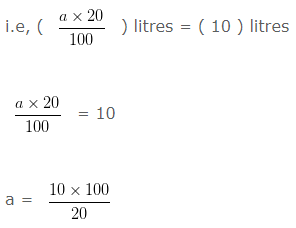]

a = 50

Hence, 10 litres is 50 % of 20 litres

Question 3:

What percent of 100 m is 80 m?

Solution:

Let a % of 100 m = 80 m

Step 1 – Convert the Percentage into Fraction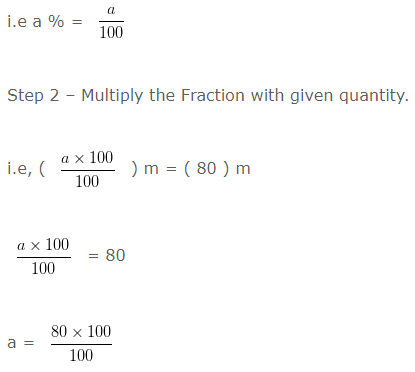a = 80

Hence, 80 m is 80 % of 100 m

Question 4:

What percent of 70 kg is 49 kg?

Solution:

Let a % of 70 kg = 49 kg

Step 1 – Convert the Percentage into Fraction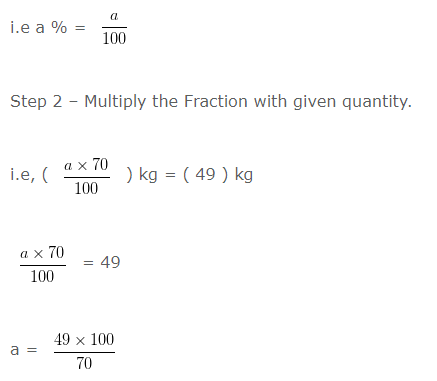a = 70

Hence, 49 kg is 70 % of 70 kg

#### Percentage Problems With Solutions – Net Increase or Decrease Percentage in a Number

Question 5:

A number is increased by 25 % and then decreased by 8 %. The net increase or decrease percentage is?

Solution:

We can form a linear equation to express the given statement and then arrive at the number

Let the required number be “a”

Step I:

If the number is increased by 25 % it would be equal to ( Given Number + 25 % of Given Number )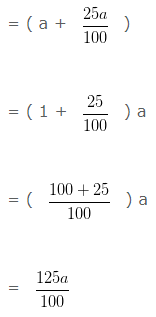Step II:

If the number at Step 1 is decreased by 8 % it would be equal to ( Increased Number – 8 % of Increased Number )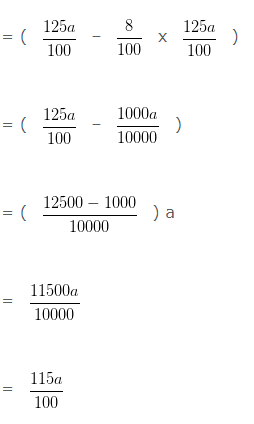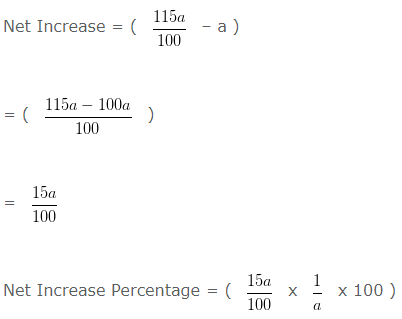= 15 %

Hence, Net Increase Percentage is = 15 %

#### Percentage Problems With Solutions – Finding a number when its decreased percentage is given

Question 6:

A number when decreased by 20% gives 160. The number is?

Solution:

Here we take the help of linear equation. First we will form the equation.

Let the number be ‘a’

20 % of ‘a’ = 20a/100

Since, the equation is:Hence, the number is 200

#### Percentage Problems With Solutions – Finding a number when its increased percentage is given

Question 7:

A number, when increased by 25% gives 375. The number is?

Solution:

The problem can be solved using linear equation.

Let the number be ‘a’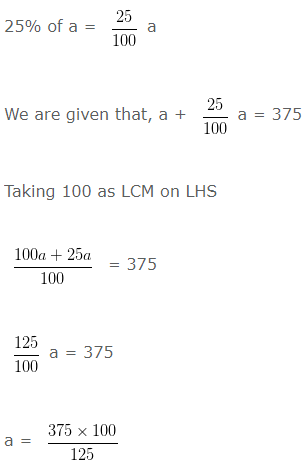a = 300

Hence, the number is 300

#### Percentage Problems With Solutions – Salary saved

Question 8:

Shruti spends 60 % of her Salary and saves the rest If she saves ₹ 5960 per month.

Solution:

Let Shruti’s monthly salary be ₹ a

Expenditure = 60 % of her Salary

Expenditure = 60 % of ₹ a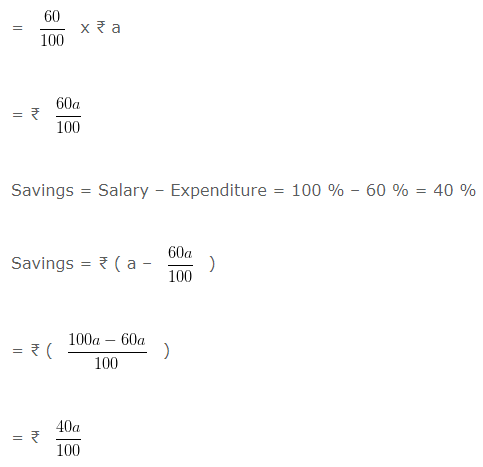Given,

Savings = 5960 = 40 % of a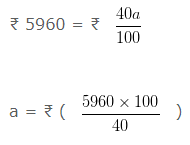a = ₹ 14900

Shruti’s monthly salary is ₹ 14900